## Speed of a Travelling wave

Wave velocity or phase velocity: The distance covered by a wave in the direction of its propagation per unit time is called the wave velocity.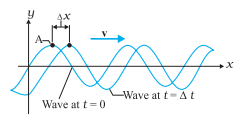Fig. Plot of a harmonic wave at t = 0 and t = ∆t.

On the waveform retains its displacement as it moves, so this is possible only when the argument is constant. It, therefore, follows that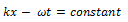Differentiating, both side w.r.t., time t, we get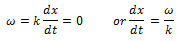But dx/dt = wave velocity, v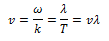### Speed of a Transverse Wave on Stretched String

The speed of transverse waves on a string is determined by two factors, (i) the linear mass density or mass per unit length, μ, and (ii) the tension T.

The linear mass density, μ, of a string is the mass m of the string divided by its length l. Therefore its dimension is [ML-1]. The tension T has the dimension of force — namely, [M L T-2].

Our goal is to combine μand T in such a way as to generate v [dimension (L T-2)].

Therefore, if v depends only on T and μ, the relation between them must be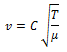Here, C is a dimensionless constant that cannot be determined by dimensional analysis.

The speed of transverse waves on a stretched string is, therefore, given by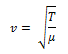The speed of a wave along a stretched ideal string depends only on the tension and the linear mass density of the string and does not depend on the frequency of the wave.

Speed of a Longitudinal Wave Speed of Sound

In a longitudinal wave, the constituents of the medium oscillate forward and backward in the direction of propagation of the wave. We have already seen that the sound waves travel in the form of compression and rarefactions of the small volume element of air. The property that determines the extent to which the volume of an element of a medium change when the pressure on it changes, is the bulk modulus B,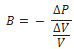Here, ∆V/V is the fractional change in volume produced by a change in pressure ∆P.

The SI unit for pressure is N m-2 pr pascal(Pa).

The dimension of density is [ML-3].

Thus, the dimension of the ratio B/ρ is [L2 T-2].

Therefore, on the basis of dimensional analysis, the most appropriate expression for the speed of longitudinal waves in a medium is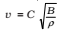where C is a dimensionless constant and can be shown to be unity.

Thus the speed of longitudinal waves in a medium is given by,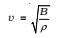The speed of propagation of a longitudinal wave in a fluid, therefore, depends only on the bulk modulus and the density of the medium. It can be shown that the speed of a longitudinal wave in the bar is given by,The relation between pressure P and volume V isWhere N is the number of molecules in volume V, kB is the Boltzmann constant and T the temperature of the gas (in Kelvin).

Therefore, for an isothermal change it follows from above eq. that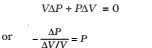Hence, substituting in eq. the speed of longitudinal wave equation,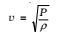This relation was first given by Newton and is known as Newton’s formula.